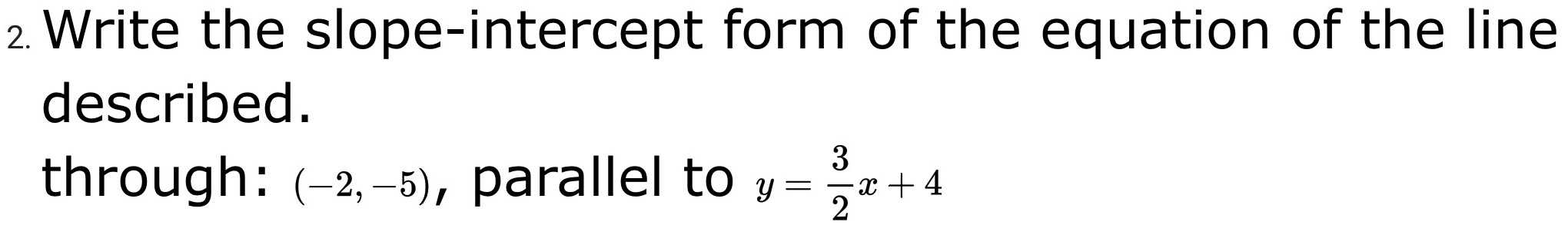### ¿Todavía tienes preguntas de matemáticas?

Pregunte a nuestros tutores expertos
Algebra
Pregunta2. Write the slope-intercept form of the equation of the line described. through: $$( - 2 , - 5 ) ,$$ parallel to $$y = \frac { 3 } { 2 } x + 4$$

$$y= \frac{3}{2}x- 2$$